SSC CGL Previous Year Questions: Time, Speed & Distance (Boat and Streams) - 1

# SSC CGL Previous Year Questions: Time, Speed & Distance (Boat and Streams) - 1 - SSC CGL

Test Description

## 20 Questions MCQ Test SSC CGL (Tier - 1) - Previous Year Papers (Topic Wise) - SSC CGL Previous Year Questions: Time, Speed & Distance (Boat and Streams) - 1

SSC CGL Previous Year Questions: Time, Speed & Distance (Boat and Streams) - 1 for SSC CGL 2023 is part of SSC CGL (Tier - 1) - Previous Year Papers (Topic Wise) preparation. The SSC CGL Previous Year Questions: Time, Speed & Distance (Boat and Streams) - 1 questions and answers have been prepared according to the SSC CGL exam syllabus.The SSC CGL Previous Year Questions: Time, Speed & Distance (Boat and Streams) - 1 MCQs are made for SSC CGL 2023 Exam. Find important definitions, questions, notes, meanings, examples, exercises, MCQs and online tests for SSC CGL Previous Year Questions: Time, Speed & Distance (Boat and Streams) - 1 below.
Solutions of SSC CGL Previous Year Questions: Time, Speed & Distance (Boat and Streams) - 1 questions in English are available as part of our SSC CGL (Tier - 1) - Previous Year Papers (Topic Wise) for SSC CGL & SSC CGL Previous Year Questions: Time, Speed & Distance (Boat and Streams) - 1 solutions in Hindi for SSC CGL (Tier - 1) - Previous Year Papers (Topic Wise) course. Download more important topics, notes, lectures and mock test series for SSC CGL Exam by signing up for free. Attempt SSC CGL Previous Year Questions: Time, Speed & Distance (Boat and Streams) - 1 | 20 questions in 20 minutes | Mock test for SSC CGL preparation | Free important questions MCQ to study SSC CGL (Tier - 1) - Previous Year Papers (Topic Wise) for SSC CGL Exam | Download free PDF with solutions
 1 Crore+ students have signed up on EduRev. Have you?
SSC CGL Previous Year Questions: Time, Speed & Distance (Boat and Streams) - 1 - Question 1

### A man goes from C to D at 40 km/h and he returns from D to C at x km/h. If the average speed of the man for the whole journey is 60 km/h, then what is the value of x?    (SSC MTS 2018)

Detailed Solution for SSC CGL Previous Year Questions: Time, Speed & Distance (Boat and Streams) - 1 - Question 1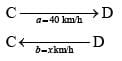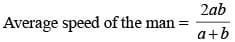We have given the average speed of the man = 60 km/h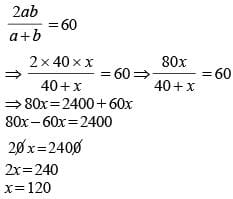SSC CGL Previous Year Questions: Time, Speed & Distance (Boat and Streams) - 1 - Question 2

### A train starts from A at 6 AM and reaches B at 11 AM on the same day. Another train starts from B at 8 AM and reaches A at 3 PM on the same day. At what time the two trains will have crossed each other?    (SSC MTS 2018)

Detailed Solution for SSC CGL Previous Year Questions: Time, Speed & Distance (Boat and Streams) - 1 - Question 2

Le the distance between A to B is d km.
Time of travel of first train = 11 – 6 = 5 hr.
Time of travel of second train = 3 p.m. – 8 a.m. = 7 hr.
then Speed of first train = d/5 km/hr..
Speed of second train = d/7 km/hr..
Now, again we suppose that two train meet after t hr. from 8 a.m.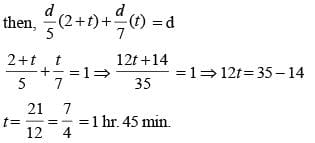Hence, time when two train meets = 8 + (1 hr 45 min) = 9:45 am.

SSC CGL Previous Year Questions: Time, Speed & Distance (Boat and Streams) - 1 - Question 3

### Walking at 7/9 of his usual speed, a person reaches his office 10 minutes later than the usual time. His usual time in minutes is: (SSC CGL-2018)

Detailed Solution for SSC CGL Previous Year Questions: Time, Speed & Distance (Boat and Streams) - 1 - Question 3

Let usual time is t minute and his speed is s m/min. then ATQ,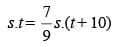9t = 7 (t + 10) 2t = 70
⇒ t = 35 minute

SSC CGL Previous Year Questions: Time, Speed & Distance (Boat and Streams) - 1 - Question 4

A train without stoppage travels with an average speed of 50 km/h, and with stoppage, it travels with an average speed of 40 km/h. For how many minutes does the train stop on an average per hour?    (SSC CGL-2018)

Detailed Solution for SSC CGL Previous Year Questions: Time, Speed & Distance (Boat and Streams) - 1 - Question 4

Distance travelled by the train in one h our without stoppage = 50 km with stoppage = 40 km
Extra distance = 50 – 40 = 10 km
Hence, stoppage time in one hour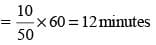SSC CGL Previous Year Questions: Time, Speed & Distance (Boat and Streams) - 1 - Question 5

Given that lengths of the paths of a ball thrown with different speeds by two boys are the same, and that the average speed for the first and second throws are respectively 90 km/h  and 162 km/h, then what is the time taken by the first throw to cover the length if the same for the second thrown is one second?    (SSC CHSL-2018)

Detailed Solution for SSC CGL Previous Year Questions: Time, Speed & Distance (Boat and Streams) - 1 - Question 5

We know that length = speed × time
For same length,
s1 × t1 = s2 × t2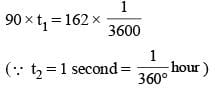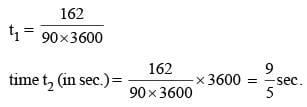SSC CGL Previous Year Questions: Time, Speed & Distance (Boat and Streams) - 1 - Question 6

A boat can go 30 km downstream and 24 km upstream in 2 hours 27 minutes. Also, it can go 10 km downstream and 4 km upstream in 37 minutes. What is the speed of the boat upstream (in km/h)?    (SSC Sub. Ins. 2018)

Detailed Solution for SSC CGL Previous Year Questions: Time, Speed & Distance (Boat and Streams) - 1 - Question 6

Let boat speed is u km/hr and stream speed = v km/hr
ATQ,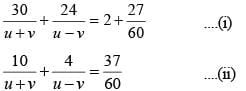Multiply equation (ii) by 3 and subtract from (i),SSC CGL Previous Year Questions: Time, Speed & Distance (Boat and Streams) - 1 - Question 7

If a train runs with the speed of 48 km/h, it reaches its destination late by 12 minutes. However, if its speed in 64 km/h it is late by 3 minutes only. The right time for the train to cover its journey (in minutes) is:    (SSC Sub. Ins. 2018)

Detailed Solution for SSC CGL Previous Year Questions: Time, Speed & Distance (Boat and Streams) - 1 - Question 7

Let the right time to reach the destination is t hr.
Then, ATQ,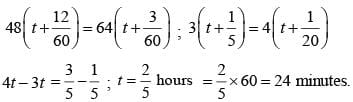SSC CGL Previous Year Questions: Time, Speed & Distance (Boat and Streams) - 1 - Question 8

A bus starts running with the initial speed of 33 km/hr and its speed increases every hour by certain amount. If it takes 7 hours to cover a distance of 315 km, then what will be hourly increament (in km/hr) in the speed of the bus?    (SSC Sub. Ins. 2017)

Detailed Solution for SSC CGL Previous Year Questions: Time, Speed & Distance (Boat and Streams) - 1 - Question 8

Here,
Sn = 315, n = 7, a = 33,  d = ?
According to A.P.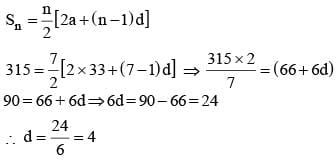∴ Required hourly increment (in km/hr) in the speed of bus = 4 km/hr.

SSC CGL Previous Year Questions: Time, Speed & Distance (Boat and Streams) - 1 - Question 9

A motorcyclist left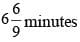later than the scheduled time but in order to reach its destination 21km away in time, he had to increase his speed by 12 km/hr from the usual speed. What is usual speed (in km/hr) of the motorcyclist?

Detailed Solution for SSC CGL Previous Year Questions: Time, Speed & Distance (Boat and Streams) - 1 - Question 9

Let usual speed of the motorcyclist = x
According to question,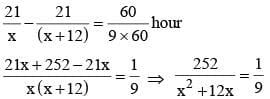⇒ x2 + 12x = 2268 ⇒ x2 + 12x – 2268 = 0
⇒ x2 + 54x – 42x – 2268 = 0 ⇒ x (x + 54) – 42 (x + 54)
⇒ (x – 42) (x + 54)
∴ x – 42 = 0 ∴ x = 42
x + 54 = 0 ∴ x =  –54
∴ Usual speed of motorcyclists = 42 km/hr.

SSC CGL Previous Year Questions: Time, Speed & Distance (Boat and Streams) - 1 - Question 10

A train covers a distance of 12 km in 10 minutes. If it takes 6 seconds to pass a telegraph post, then the length of the train is:    (SSC MTS 2017)

Detailed Solution for SSC CGL Previous Year Questions: Time, Speed & Distance (Boat and Streams) - 1 - Question 10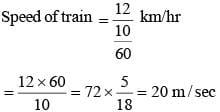∴ Length of train = speed × time = 20 × 6 = 120 m.

SSC CGL Previous Year Questions: Time, Speed & Distance (Boat and Streams) - 1 - Question 11

If a train maintains an average speed of 42 km per hour, it arrives at its destination at the right time, if however, the average speed is 40 km per hour, it arrives 15 minutes late. Find the length of the journey?    (SSC MTS 2017)

Detailed Solution for SSC CGL Previous Year Questions: Time, Speed & Distance (Boat and Streams) - 1 - Question 11

Let Length of Journey = x km.
According to question,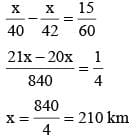∴ Length of Journey is = 210 km

SSC CGL Previous Year Questions: Time, Speed & Distance (Boat and Streams) - 1 - Question 12

Two cars travel from city A to city B at a speed of 36 km/hr and 48 km/hr respectively. If one car takes 3 hours lesser time than the other car for the journey, then the distance between City A and City B is    (SSC CHSL 2017)

Detailed Solution for SSC CGL Previous Year Questions: Time, Speed & Distance (Boat and Streams) - 1 - Question 12

According to question,
Speed of cars = 36 km/hr and 48 km/hr
So, 36 × t = 48 (t – 3)
36t = 48t – 48 × 3
12t = 48 × 3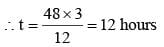∴ Distance = 12 × 36 = 432 km.

SSC CGL Previous Year Questions: Time, Speed & Distance (Boat and Streams) - 1 - Question 13

A bus starts running with the initial speed of 21 km/hr and its speed increases every hour by 3 km/hr. How many hours will it take to cover a distance of 252 km?    (SSC CGL 2017)

Detailed Solution for SSC CGL Previous Year Questions: Time, Speed & Distance (Boat and Streams) - 1 - Question 13

Since speed of bus increases every hour by 3 km/hr.
∵ Initial speed = 21 km/hr
Total distance = 252 km
According to Arithmetic Progression
a = 21, d = 3, sn = 252 n = ?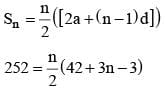504 = n(3n+ 39)
3n2 + 39n – 504 = 0
n2 + 21n – 8n – 168 = 0
⇒ n(n + 21) – 8 (n + 21) = 0
∴ (n – 8) (n + 21)
⇒ n = 8, n ≠ – 21
∴ So, 8 hours will it take to cover a distance of 252 km.

SSC CGL Previous Year Questions: Time, Speed & Distance (Boat and Streams) - 1 - Question 14

A train leaves Delhi at 10 a.m. and reaches Jaipur at 4 p.m. on same day. Another train leaves Jaipur at 12 p.m. and reaches Delhi at 5 p.m. on same day. What is the time of day (approximately) when the two trains will meet?    (SSC CGL 2017)

Detailed Solution for SSC CGL Previous Year Questions: Time, Speed & Distance (Boat and Streams) - 1 - Question 14

Suppose distance between Delh i and Jaipur is 30 km.
Then, Speed of first train = 30/6 = 5 km/hr
Speed of second train 30/5 = 6 km/hr
If trains met after t hours from 10 a.m. then
5t + 6 × (t – 2) = 30 ⇒ 5t + 6t – 12 = 30 11t = 42
t = 42/11 hours = 3 hours 49 minutes
∴ Trains meet 3 hours 49 minutes after 10 a.m. i.e. at 1:49 pm.

SSC CGL Previous Year Questions: Time, Speed & Distance (Boat and Streams) - 1 - Question 15

A train travels 40% faster than a car. Both start from point A at the same time and reach point B, 140 km away at the same time. On the way the train takes 25 minutes for stopping at the stations. What is the speed (in km/hr) of the train?    (SSC CGL 2017)

Detailed Solution for SSC CGL Previous Year Questions: Time, Speed & Distance (Boat and Streams) - 1 - Question 15

Let speed of car = x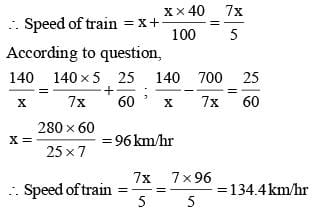SSC CGL Previous Year Questions: Time, Speed & Distance (Boat and Streams) - 1 - Question 16

A boat goes 15 km upstream and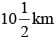downstream in 3 hours 15 minutes. It goes 12 km upstream and 14 km downstream in 3 hours. What is the speed of the boat in still water?    (SSC CGL 2017)

Detailed Solution for SSC CGL Previous Year Questions: Time, Speed & Distance (Boat and Streams) - 1 - Question 16

Let speed of the boat in still water be x km/h and speed of current be y km/h.
Then,
upstream speed = (x – y) km/h
and downstream speed = (x + y) km/h
Now,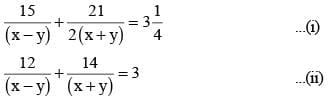From Equation (i) and (ii)
x = 10 km/hr and y = 4 km/hr.

SSC CGL Previous Year Questions: Time, Speed & Distance (Boat and Streams) - 1 - Question 17

A man travelled a distance 72 km in 12 hour. He travelled partly on foot at 5 km/hour and partly on bicycle at 10 km/ hour. The distance travelled foot is    (SSC Sub. Ins. 2016)

Detailed Solution for SSC CGL Previous Year Questions: Time, Speed & Distance (Boat and Streams) - 1 - Question 17

Let distance travelled by foot = x km/hr
Let distance travelled by bicycle = 72 – x km/hr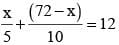2x + 72 – x = 120
x = 120 – 72 = 48 km

SSC CGL Previous Year Questions: Time, Speed & Distance (Boat and Streams) - 1 - Question 18

Points A and B are 100 km apart on a highway. One car starts from A and another from B at the same time. If the cars travel in the same direction, they meet in 5 hours. If the cars travel towards each other, they meet in 1 hour. What is the speed of the faster car?    (SSC Sub. Ins. 2016)

Detailed Solution for SSC CGL Previous Year Questions: Time, Speed & Distance (Boat and Streams) - 1 - Question 18

Let the speed of car be x and other be y
Distance covered from A in 5 hrs = 5x
Distance covered from B in 5 hrs = 5y
ATQ
when they travel in same direction then 5x – 5y = 100
x – y = 20 ...(i)
When they travel towards each other then
ATQ x + y = 100 ...(ii)
Now, adding eqn. (i), & (ii)
2x = 120
x = 60 km/hr

SSC CGL Previous Year Questions: Time, Speed & Distance (Boat and Streams) - 1 - Question 19

A train, 200 m long, is running at a speed of 54 km/hr. The time in seconds that will be taken by train to cross a 175 m long bridge is    (SSC CGL 1st Sit. 2016)

Detailed Solution for SSC CGL Previous Year Questions: Time, Speed & Distance (Boat and Streams) - 1 - Question 19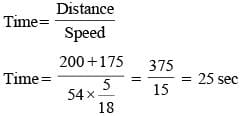SSC CGL Previous Year Questions: Time, Speed & Distance (Boat and Streams) - 1 - Question 20

A man covers a total distance of 100 km on bicycle. For the first 2 hours, the speed was 20 km/hr and for the rest of the journey, it came down to 10 km/hr. The average speed will be    (SSC CGL 1st Sit. 2016)

Detailed Solution for SSC CGL Previous Year Questions: Time, Speed & Distance (Boat and Streams) - 1 - Question 20

Total Distance = 100 km
Distance travelled in first 2hr = 2 × 20 = 40 km
Remaining  distance = 100 – 40 = 60 km
Time taken = 60/10 = 6 hr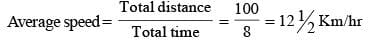## SSC CGL (Tier - 1) - Previous Year Papers (Topic Wise)

250 tests
Information about SSC CGL Previous Year Questions: Time, Speed & Distance (Boat and Streams) - 1 Page
In this test you can find the Exam questions for SSC CGL Previous Year Questions: Time, Speed & Distance (Boat and Streams) - 1 solved & explained in the simplest way possible. Besides giving Questions and answers for SSC CGL Previous Year Questions: Time, Speed & Distance (Boat and Streams) - 1, EduRev gives you an ample number of Online tests for practice

250 tests